## Contracting the Indices

Suppose we have a tensor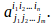To contract any two indices is equate any two indices, thus summing over those indices.

If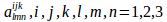is a tensor then we can contract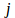and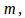writing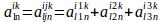If there are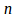indices in the supersript and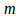indices in the subscipt, with each index able to take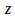values then contracting one pair of indices will reduce the total order of the system from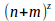to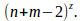If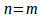then we can contract the idices completely to give a sum ofterms.